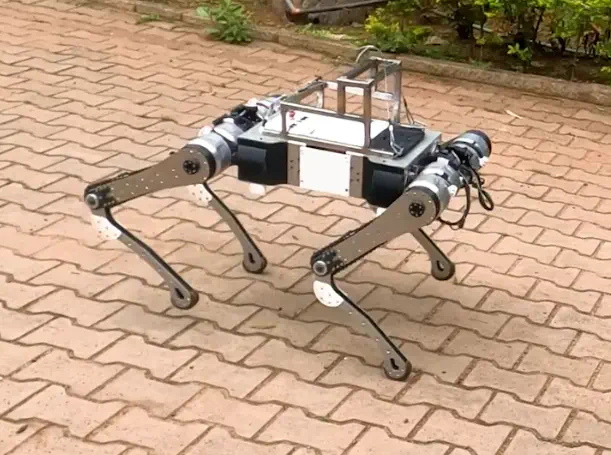# Representation Free Model Predictive Control for Legged LocomotionModel Predictive Control is a popular and powerful control algorithm used in legged robot locomotion. In this project we show the application of the recently published walking controller, Representation Free Model Predictive Control (RF-MPC), to a quadruped robot, Stoch-3, in a simulation environment. The RF-MPC controller directly represents the rotational dynamics using the rotation matrix. Other walking controllers like Convex-MPC use Euler angles for representing orientations, which have singularity and approximation issues. RF-MPC models the quadruped robot as a single rigid body with massless legs. It uses a variation-based linearization scheme to linearize the model. The MPC control law is written in a standard Quadratic Program (QP) form.

The Algorithm can perform step-in-place motion for some duration after which the solution starts to diverge and the robot becomes unstable. The main reason behind this is the suboptimal reference trajectory of control inputs based on simple heuristics. Hence we generate the reference trajectory of control inputs for the RF-MPC by using the Convex MPC algorithm at a lower frequency. With this, we have been able to achieve robust performance for all different types of gaits. The Convex MPC runs at 30 Hz and RF-MPC runs at 300 Hz. We demonstrate in-place stepping, forward walking, in-place turning, and sideways walking using the trot gait on the Stoch-3 robot in the Pybullet simulation environment. We also performed some preliminary hardware experiments for sim2real validation